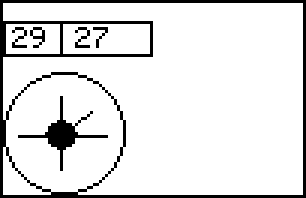RicScript

RicScript is a text base method for defining RIC files and was developed by John. C. Hansen.

With RicScript you can create the same kind of RIC files like you can do it with nxtRICeditV2 - but the big difference is that you have to use a text based editor for defining the RicScript files. The syntax of RicScript isn't very complex and will be described as following:

Element Definition

Description

desc( options, width, height );

In the current version of the firmware the paramter options will not be used and should be 0. The other two parameters will only be used by NXT-G - so the Description element has no effect for drawing pictures on the LCD

Example:

 desc( 0, 50, 20 );

Sprite

sprite( id, bitfields );

sprite( id, hexfields );

sprite( id, import( filename ) );

sprite( id, import( filename, brightness-threshold ) );

sprite( id, import( filename, brightness-threshold, destination_width, destination_height ) );

Define a Sprite element. You can define it with direct information about the pattern or you can import an existing gaphic file.

Example 1:

 sprite( 1, 11111111,            10000001,            10000001,            11111111  );

Example 2:

 sprite( 2, 0xFF,            0x81,            0x81,            0xFF  );

Example 3:

 sprite( 2, import( "c:\temp\picture.bmp", 50, 80,40 );

VarMap

varmap( id, interpolation-point-definitions );

Define a interpolation function which can be used to transfer given parameter values to a destination value. Domain value of an interpolation point is between 0 and 255. The range value must be between 0 and 65535. Minimum count of interpolation points is 2 - maximum count of interpolation point is 256.

Example:

 varmap( 4, f(3)=10,            f(10)=0,            f(20)=200,            f(50)=100 );

CopyBits

copybits( copyopt, spriteid, source_x, source_y, width, height, dest_x, dest_y );

CopyBits will be used for transfering a rectangle area from the addresed sprite to the screen. Parameter copyopt is currently not used and should be 0.

Example:

 copybits( 0, 1, 10, 10, 30, 20, 0, 0 );

Pixel

pixel( copyopt, pos_x, pos_y );

pixel( copyopt, pos_x, pos_y, value );

The Pixel element can be used for drawing a single point on the NXT display. Parameter value is optional and will not be used in the current firmware version. Parameter copyopt is also not supported by the current firmware and should be set to 0.

Example:

 pixel( 0, arg( 0 ), maparg( 4,0 ) );

Line

line( copyopt, start_x, start_y, end_x, end_y );

Draw a line from the start point (start_x/start_y) to the end point (end_x/end_y). Parameter copyopt isn't supported by the current firmware and should be set to 0.

Example:

 line( 0, 10, 20, 60, 25 );

Rectangle

rect( copyopt, edge1_x, edge1_y, edge2_x, edge2_y );

Draw a rectangle which is defined by the 2 oposite points (edge1_x/edge1_y) and (edge2_x/edge2_y). Parameter copyopt isn't supported by the current firmware and should be set to 0.

Example:

 rect( 0, 10, 20, 60, 25 );

Circle

circle( copyopt, center_x, center_y, radius );

Draw a circle with center point (center_x/center_y) and the given radius. Parameter copyopt isn't supported by the current firmware and should be set to 0.

Example:

 circle( 0, 50,32, 30 );

NumBox

numbox( copyopt, pos_x, pos_y, value );

Display the given value as a number on the NXT screen on the given position (pos_x/pos_y). Please keep in mind that the vertical position pos_y will be snaped to one of the 8 possible text lines. Parameter copyopt isn't supported by the current firmware and should be set to 0.

Example:

 numbox( 0, 0, 16, arg( 0 ) );

Example for a RicScript file which shows following output:

 Output on the NXT screenRicScript: desc( 0, 51, 51 ); circle( 0, 20, 20, 20 ); varmap( 1,         f( 0)= 0,         f( 16)= 35,         f( 42)= 18,         f( 53)= 7,         f( 71)= 17,         f( 76)= 39,         f( 99)= 63,         f( 112)= 23,         f( 178)= 12,         f( 205)= 35 ); sprite( 2,         000000000000001000000000000000,         000000000000001000000000000000,         000000000000001000000000000000,         000000000000001000000000000000,         000000000000001000000000000000,         000000000000001000000000000000,         000000000000001000000000000000,         000000000000001000000000000000,         000000000000011100000000000000,         000000000001111111000000000000,         000000000011111111100000000000,         000000000011111111100000000000,         000000000111111111110000000000,         111111111111111111111111111111,         000000000011111111100000000000,         000000000011111111100000000000,         000000000001111111000000000000,         000000000000011100000000000000,         000000000000001000000000000000,         000000000000001000000000000000,         000000000000001000000000000000,         000000000000001000000000000000,         000000000000001000000000000000,         000000000000001000000000000000,         000000000000001000000000000000 ); copybits( 0, 2, 0, 0, 30, 25, 5, 8 ); numbox( 0, 24, 54, maparg( 1, 0 ) ); numbox( 0, 3, 51, arg(0) ); line( 0, 19, 46, 19, 56 ); line( 0, 20, 20, arg(0), maparg( 1, 0 ) ); rect( 0, 0, 46, 49, 11 );